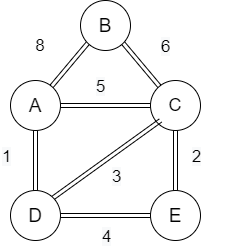# Yen's k-Shortest Path Algorithm in Data Structure

Instead of giving a single shortest path, Yen’s k-shortest path algorithm gives k shortest paths so that we can get the second shortest path and the third shortest path and so on.

Let us consider a scenario that we have to travel from place A to place B and there are multiple routes available between place A and place B, but we have to find the shortest path and neglect all the paths that are less considered in terms of its time complexity in order to reach the destination.

Let us understand with an example-Consider the given example as the bridge which is having a peak of B. If someone wants to cross the bridge from A to C, then nobody will go to the peak to cross the bridge. So it would be a bit longer path from A to C.

There are multiple ways to get the shortest path. But we have to find the shortest path up to (k-1).

## Algorithm for k-shortest Path

query= “””
MATCH(start: place{id:source}),*end: Place {Id:destination})
Call algo.kshortestPaths.stream(start,end,10, “distance”)
Yield nodeIDs, path costs, index
Return index.
[node in algo.getNodeByID(nodeId[1…..-1]) | node.id] aS,
Reduce (acc=0.0, cost in costs | acc+cost ) as total cost
“””
params= {“source”: Alex,Destination: “US”}
With driver.selection() as session:
Row session.run(query, params)
df = pd.DataFrame[dict(record) for record in rows])
pd.set_option(‘max_colwidth’, 100)
display(df)